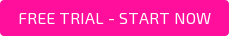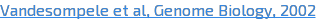### The gene expression blog

Most quantitative PCR (qPCR) results in journals are nowadays presented as Ct, delta Ct (dCt) or delta delta Ct (ddCt) values. Often the correct interpretation of the Y-axis in graphs has to be derived from the numbers given, which are not always in line with the axis title. This title most probably indicates that the axis represents the fold difference of the normalized expression of the target gene between the experimental and control conditions. It is of note that by now the 2 and the minus sign that should be there to turn a delta delta Ct into such a fold-difference have disappeared almost completely from publications. This tendency to avoid formulas has turned quantitative PCR into a field of meaningless numbers. For references to illustrate this, just google delta delta Ct.

Although this situation gives the impression that delta delta Ct needs no explanation for the users of qPCR, the opposite is true. Seemingly the presence of asterisks (to denote statistical significance) in the graphs satisfies the reviewer and the reader and thus abolishes the need for further understanding; the results are significant whatever they are. Without knowing, the reader has assumed that the amplification efficiencies of both target of interest and reference gene were all equal to 2, which means a doubling per PCR cycle or a 100% efficient assay. However, from the onset of qPCR, the authors in the field have highlighted that dCt and ddCt cannot be applied when there is a difference in amplification efficiency of the target and reference genes being compared.

The 2-ddCt equation for relative quantification that is referred to in most publications was originally accompanied by a procedure to check that the amplification efficiencies of the target and reference genes were sufficiently similar (1). However, within a few years of qPCR it was clear that the implicit assumption of this equation that all PCR assays were 100% efficient was wrong and an alternative equation for efficiency corrected relative quantification was published (2). The required PCR efficiency could be derived from a dilution series. When such dilution series are dutifully performed, the linearity of the Ct against log(input) is checked and, if confirmed, the resulting PCR efficiencies are quietly forgotten. Indeed, my experience is that in qPCR courses, less than 50% of the participants is aware of the role of the amplification efficiency in PCR.

Equation alert: skip the next paragraph when you are allergic to equations. However, reading this paragraph will make it easier to understand why the amplification efficiency cannot be ignored.

As the MIQE guidelines () propose to use quantification cycle (Cq) over treshold cycle (Ct) and other terms referring to the same value from the real-time instrument, I'll be using Cq for the remainder of this post.

Whereas the original paper on efficiency corrected relative quantification (2) attempted to stay close to the original ddCq equation, the realization that multiple reference genes were required (3) necessitated the developers of qPCR analysis software to go back to the original kinetic equation of the PCR reaction (NC=N0.EC). The target quantity N0 can be directly derived from inversion of this equation and the definition of Cq. Because Cq is the number of cycles needed to reach a threshold Nq, the inversion of the kinetic equation shows that N0=Nq/ECq (4). When the Cq is derived for a target gene (with efficiency ET) and for a reference gene (ER), for control and treated conditions, the relative gene expression (target/reference for treated/control) can be calculated as Fold= E_R^((C_(q,R,Tr)-C_(q,R,C) ) )/E_T^((C_(q,T,Tr)-C_(q,T,C) ) ) [Eq. 1].

With the assumption that both efficiencies are 2 and a rearrangement of the Cq values in the exponent this equation ‘simplifies’ to Fold= 2^(-((C_(q,T,Tr)-C_(q,R,Tr) )-(C_(q,T,C)-C_(q,R,C) )) ) [Eq. 2] which is the full write-out of the 2-ddCq equation.

The effect of this simplifying assumption is illustrated with four target genes and a reference gene in an experiment with three treatments and a control condition (see table and figure). The target genes all have the same expression level but show a three-fold increase between the control and each of the treatments; the expression level of the reference gene is constant in all conditions. Note that each target is amplified with another efficiency. Target 1, amplified with an efficiency of 2, shows the correct fold difference in each of the treatments, regardless whether [Eq. 1] or [Eq. 2] is used for the calculation. For treatment 1, with only a 3-fold difference compared to the control, the use of [Eq. 2] results in only slightly deviating values, even when the efficiency value of the target is far below 2. However, with increasing effect of the treatment and decreasing PCR efficiency of the targets the bias caused by [Eq. 2] becomes increasingly clear. Given the sensitivity of qPCR, with enough biological replicates the use of the ddCq equation will lead to the conclusion that the targets are differentially expressed in treatments 2 and 3. This obviously wrong conclusion illustrates why the actual PCR efficiencies have to be included in the calculation of qPCR results.

But what is the reason that PCR efficiencies of the targets and references are often ignored and implicitly considered to be 2? Probably because most researchers, reviewers and readers think that in relative quantification the efficiency differences disappear from the calculation. The example shows that is not the case. It shows what can happen to the published results when PCR assays are not 100% efficient.Figure 1: The table shows that for all targets the starting input concentrations are the same, increasing 3-fold between control and subsequent treatments. The different amplification efficiencies of the targets make that for each target and condition another number of cycles (Cq) is needed to reach the quantification threshold (in this example arbitrarily set at 0.04). The fold-difference of the normalized gene expression between treatment and control conditions is 3, 9 and 27 for all targets per treatment (blue lines, calculated with the efficiency and Cq values in the table and [Eq. 1]). If all efficiency values are assumed to be 2, the reported fold-difference between treatment and control (red lines, calculated with the Cq values in the table and [Eq. 2]) erroneously increases with decreasing PCR efficiency value and increasing true fold difference.

### References

1. Livak, K.J. and Schmittgen, T.D. (2001). Analysis of relative gene expression data using real-time quantitative PCR and the 2(-Delta Delta C(T)) method. Methods, 25, 402-408.
2. Pfaffl, M.W. (2001) A new mathematical model for relative quantification in real-time RT-PCR. Nucleic Acids Res, 29, e45.
3., 3, RESEARCH0034.
4. Ruijter, J.M., Ramakers, C., Hoogaars, W.M., Karlen, Y., Bakker, O., van den Hoff, M.J. and Moorman, A.F. (2009) Amplification efficiency: linking baseline and bias in the analysis of quantitative PCR data. Nucleic Acids Res., 37, e45.

Topics: qPCR- PCR efficiency- delta delta Ct### J.M. Ruijter

J.M. Ruijter is one of the AMC Principal Investigators. In previous years he has developed methods for 3D analysis and visualization of gene expression patterns and morphogenetic parameters during embryogenesis and for the analysis of quantitative PCR (LinRegPCR) and transcript count (SAGEstat and Gtest) data.

Previous Post

Next Post

### Stay up to date

Subscribe and we'll send new blog posts directly to your inbox!